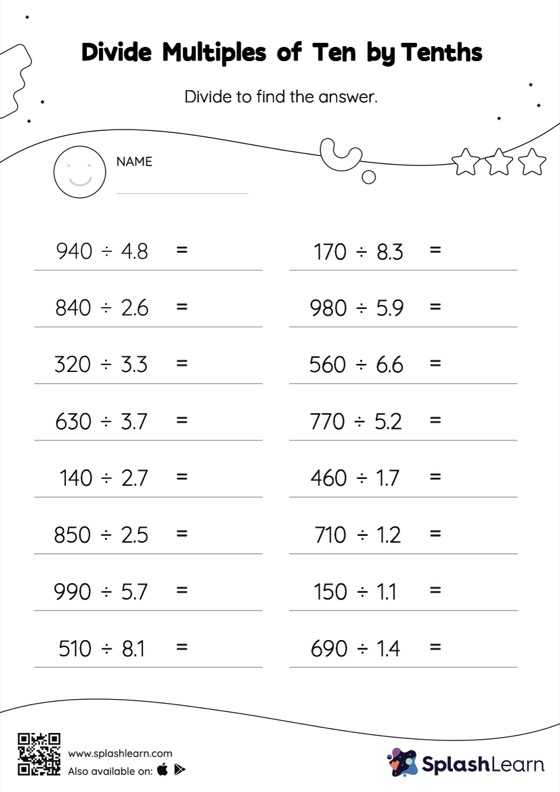# Divide Multiples of Ten by Tenths with Remainder: Horizontal Division Worksheet

Home > Divide Multiples of Ten by Tenths with Remainder: Horizontal DivisionStudents divide a number by a decimal without taking the decimal point into account, and then, depending on the number of decimal digits in the divisor, they move each digit of the quotient to the left. Students practice this concept in great depth with divide multiples of ten by tenths with remainder worksheet. As the worksheet uses a horizontal format, it allows for more creativity when it comes to how the student solves the problem. In contrast, in the vertical format, students usually employ the standard method to solve.